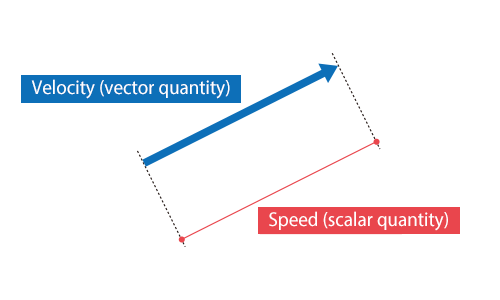# Speed

## Speed

Speed is a distance traveled per unit time. Its SI unit is [m/s].

It is a scalar quantity with only magnitude. Speed equals to magnitude of velocity.

It can be expressed with the following equation:where;
v: Veclocity [m/s]
r: Distance traveled [m]
t: Time taken [s]